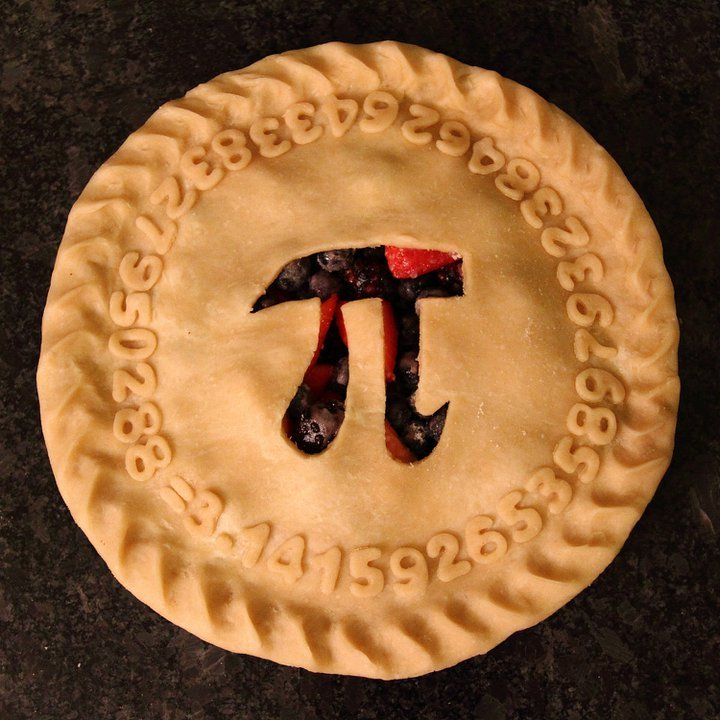# Pi Day and the Significance of Math

Pi day, a day to celebrate the irrational number and of general mathematics. Many people would eat pie, others would be a part of a pi competition and others wouldn’t do anything at all. With this in mind, I wondered to myself, what will I do for You day? My conclusion to this would be that I will reflect on math and how we use it in a daily basis. Also, I would wonder about Pi and why it has a day to itself.Pi day reminded me of my use and other persons use of math on a day-to-day basis. For me, I used trigonometric functions for my pre-calc class, but I most likely would not use it on a daily basis when it is summer vacation. There are also other students who use statistics, algebra and geometry within their respective math class. Of those three, algebra would most likely be used on a daily basis since you will be able to make basic  averages and find the area, or surface area of a simplistic two-dimensional or three-dimensional object. With this in mind though, basic addition, subtraction, multiplication and division would be the most widely used form of mathematics on a day to day basis for prices on food, how much money you will have left after buying an item, how much everyone should get form an item, and for how much of an item you will need for a certain amount of people.Pi, the ratio of a circle’s circumference to its diameter. It can used to calculate practically anything related to circles, spheres and trigonometric functions. The problem with this is that for the unit circle, there are 2π radians, a confusing choice to describe the number of radians within a circle. That is why there is another circle constant, tau. Tau is just double Pi, so we would be able to describe the circle as having tau radians. Tau could also have its own day on 6/28, so why do people still use pi? I think it is based off of the fact that π has history to it while tau is a recent proposal. So this is what Pi day is about, a historical constant to show the importance of mathematics in our day-to-day lives.## 2 thoughts on “Pi Day and the Significance of Math”

1.Lee Ann Spillane says:

That is a gorgeous Pi pie. Where did you find the image?

2.sashiiiieeee says:

I really liked this a lot nice job